Scan QR code or get instant email to install app

Question:

# 1212Which exponential equation represents this logarithmic equation?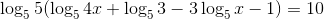A 1212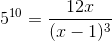explanation

1212Use the properties of logarithms to condense the given equation, then use the equivalence between logarithms and exponents to determine, which becomes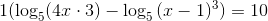, which becomes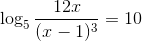Recall that a logarithm of the form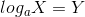can be written as an exponential equation as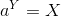Applying this to the logarithm above: